# Square

If we increase one side of square by its one-half then square perimeter increase by 10 cm.

What is the side of the square?

a =  5 cm

### Step-by-step explanation: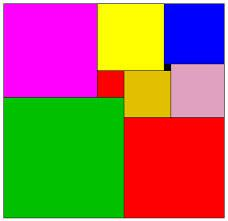Did you find an error or inaccuracy? Feel free to write us. Thank you!Tips to related online calculators
Do you have a linear equation or system of equations and looking for its solution? Or do you have a quadratic equation?

#### You need to know the following knowledge to solve this word math problem:

We encourage you to watch this tutorial video on this math problem:

## Related math problems and questions:

• Second sideCalculate the length of the other side of the rectangle if its circumference is 60 cm and one side is 10 cm long.
• Triangle ABCIn a triangle ABC side b measure 10 cm less than the side a and side b is half of the side c. Calculate the length of sides if the circumference of the triangle is 42 cm.
• Square gridSquare grid consists of a square with sides of length 1 cm. Draw in it at least three different patterns such that each had a content of 6 cm2 and circumference 12 cm and that their sides is in square grid.
• RhombusIf we increase the rhombus side by 2.4 cm; its perimeter is 20 cm. How long was the side of the rhombus?
• Square sides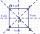If we enlarge the side of the square by a third, its circumference will be 20 cm larger than the original. What is the side of the square?
• Square and circle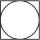Into square is an inscribed circle with diameter 10 cm. What is the difference between circumference square and circle?
• Circumscribed circle to squareFind the length of a circle circumscribing a square of side 10 cm. Compare it to the perimeter of this square.
• Do you solve this?Determine area S of rectangle and length of its sides if its perimeter is 102 cm.
• 3x square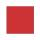The side length of the square is 54 cm. How many times increases the content area of a square if the length of side increase three times?
• Tablecloth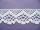The table top is 90 cm long and 50 cm wide. How many cm lace edging is necessary for the tablecloth that hangs on each side by 10 cm?
• The perimeter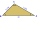The triangle has one side 5 cm long and the another 11 cm long. What can be the smallest and what is the largest perimeter?
• Perimeter of rhombus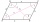The area of the rhombus is 32 cm2. One side of it measures 10 cm. The height on the other side measures 5 cm. What is the circumference of this rhombus?
• Perimeter from areaWhat is the perimeter of the square if its content is 64 cm2?
• Square sideIf we enlarge the square side a = 5m, its area will increase by 10,25%. How many percent will the side of the square increase? How many percent will it increase the circumference of the square?
• Diagonal of squareCalculate the side of a square when its diagonal is 10 cm.
• Perimeter to area 2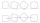Find the area of a square whose perimeter is 100 cm with solve.
• Glass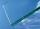Peter broke the window glass with size 110 cm and 90 cm. 1 square meter of glass costs 11 USD. How much money is need to pay for a new glass?